Power conversion circuits with control loop(s) are everywhere in electronic systems. We must establish stability and performance metrics for control loops and their circuits. However, generally accepted metrics may not be good enough. Is a crossover frequency with 45 degrees of phase margin and 10 dB of gain margin enough? How can we relate phase margin to peaking in the impedance profile and transient noise requirements? Determining which method to use for evaluating the circuit's performance, defining the respective metrics, and knowing under what circumstances are the methods and metrics valid, are important to success.

Power conversion circuits are everywhere in our electronic systems. They convert the AC line voltage to 48V or 12V DC for system-wide distribution, large and small DC-DC converters feed individual integrated circuits or clusters of loads, and low-current analog regulators feed sensitive analog circuits. A common characteristic is that (with a few exceptions) they all have control loop(s) to stabilize one or more output parameters, for instance the output voltage, or how current is shared in multi-phase and paralleled converters. For sake of simplicity, in the rest of the paper we will limit our discussions and examples to the single-phase regulated buck converter topology, where our goal is to keep the output voltage within pre-defined limits in spite of input voltage and load current transients.  Aging and temperature dependence are assumed to be handled separately by tolerance analysis.

The frequency-dependent loop gain in a DC-DC converter not only gives insight about the stability of its control loop, it is also related to other important performance metrics , .  The top sketch in Figure 1 shows the block diagram of a basic power converter with the linearized transfer functions of its blocks noted.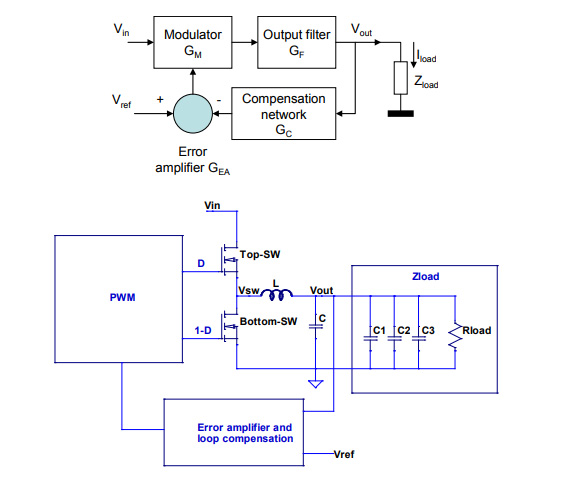Figure 1: Simplified block diagrams of a power converter with feedback loop.

The bottom sketch in Figure 1 elaborates a little on the output filter and load impedance.  The output filter is a second-order network with a series inductor L and one or more parallel capacitors, collectively denoted as C.  The load impedance is usually also complex; in most applications the system board has to have additional capacitors, here denoted as C1, C2 and C3.  These additional capacitors are needed either for improving the converter’s in-band response and/or required by the load for out-of-band high-frequency bypassing.

As we will discuss it later, in many practical circuits we also have to consider intentional or unintentional series elements, which make the output node, here denoted by a single lumped Vout net, distributed in nature.  This creates two possible complications: a) the load impedance with its capacitors is inside the feedback loop and b) when the output net becomes distributed, the position where we connect the sense line (and –in case the output impedance has its target value- the location where we measure the impedance) also becomes part of the loop equation.

By applying a linearized model to each block, we can calculate the overall loop gain around the closed feedback loop and apply the conventional stability criteria during the design and analysis processes.  By denoting the modulator gain by GM, output filter gain by GF, compensation network gain by GC and error amplifier gain by GEA, the total loop gain can be described as: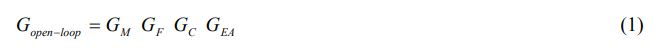All constituents of the loop-gain product can be, and usually are, frequency dependent complex numbers.  There are two major input variables that will affect the output voltage: drift and/or transients in the input voltage as well as drift and/or transients in the load current.  In a linearized equivalent circuit of the converter, the effect of input voltage variation can be described with a ΔVout/ ΔVin voltage transfer function and the effect of load current variation can be described through a ΔVout/ΔIload impedance.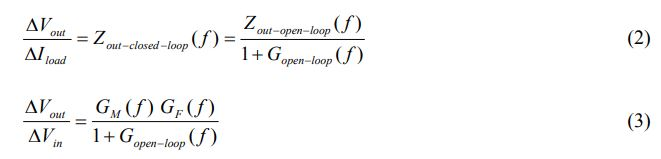The 1+Gopen-loop denominator of these expressions is called the characteristic expression.

Control feedback loops can become unstable and could ultimately self-oscillate. Therefore, we have to establish metrics to quantify how far the circuit is from self-oscillation. A commonly used metric for analog feedback loops is the Gain-phase plot (Bode plot), which depicts the complex open-loop gain as a function of frequency. An example Bode plot is shown in Figure 2

There are usually three numbers cited on a gain-phase plot with respect to the stability of control loops: cross-over frequency, phase margin, and gain margin, as they are marked in Figure 2.  The cross-over frequency is defined as the frequency where the gain magnitude first reaches unity or zero dB.  The phase at the crossover frequency is called the phase margin.  The gain magnitude at the frequency where the phase first becomes zero is called the gain margin.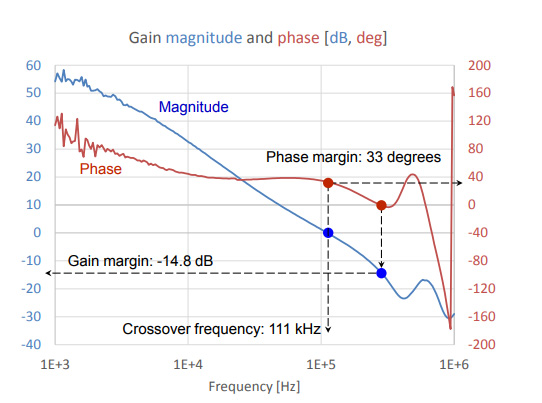Figure 2: An example Gain-phase (Bode) plot of a DC-DC converter with the cross-over frequency, phase margin and gain margin values marked.

It is customary to mandate a minimum phase margin of 45 degrees and a gain margin of 10 dB.  By these customary requirements, the illustration in Figure 2 exhibits fairly poor phase margin but decent gain margin.  Different applications may specify the minimum margin numbers differently: conservative designs may mandate to have at least the specified minimum margin under all operating conditions. Some designs validate and require the margin numbers to be met at a few operating points only.

Phase margin and gain margin based on the gain-phase plots are useful and safe for monotonic drop of gain magnitude with 20 dB/decade or higher slopes, but will not tell us enough about the loop stability in cases where the frequency dependencies are more complex.  This often happens with Type III compensation and/or control loops with digital signal processing .  Gain and phase margins can be measured directly  or indirectly .  In fact there are several approximations available for indirect measurements of Bode plots as we will show it later in the paper.

Another possible form to express loop stability is the Nyquist plot, which uses the same data as the gain-phase Bode plot, except it is presented in a different visual form.  The gain-phase plots show the gain magnitude and phase of the gain as two separate traces as a function of frequency.  The Nyquist plot uses the real and imaginary parts of the complex loop gain and plots them on the complex plane with real part being on the horizontal scale and imaginary part being on the vertical scale.  Frequency is the parameter along the curve.  As an illustration, Figure 3 plots the data from Figure 2 in a Nyquist plot form in the 30 – 300 kHz frequency range (blue trace). The green line shows the unity circle around the critical point and it represents the operating points where the loop-gain magnitude is unity.  The blue trace crosses the green circle at the crossover frequency and the phase of that point is the negative phase margin.  The gain value where the blue trace crosses the horizontal axis is the gain margin value.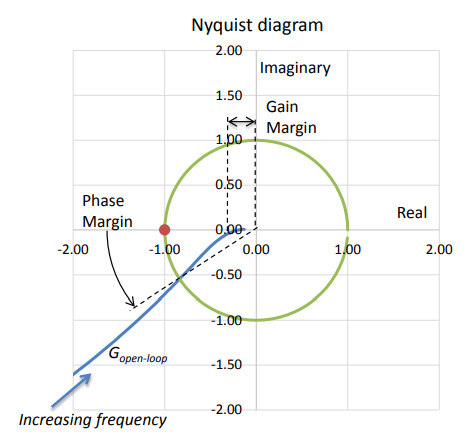Figure 3: Nyquist plot.

To capture the risk of instability in a wider frequency range, stability margin can also be used, which shows the distance of the operating point from the critical point of -1.  We can do this by plotting the 1 + Gopen-loop characteristic expression or the inverse of it.  The magnitude of the inverse of the characteristic expression tells us by how much the active control loop changes the open-loop output impedance, potentially increasing it, instead of decreasing.  Figure 4 is a combined plot of the characteristic expression (red trace) and its inverse (blue trace) for the data from Figure 2.  A simple quality check from Equation (2): wherever the inverse of the characteristic impedance is bigger than one, the OFF impedance will be pushed up, instead of being pushed down, by the active loop.

While maintaining stability is the first and most important requirement, it is not the only one we have to satisfy.  The power distribution network (PDN) usually has a requirement to keep the voltage for the load(s) within predefined limits in spite of changes in load current, input voltage, temperature, component tolerances, and aging.  This requirement is also a must, but unfortunately the fact that the regulator is stable has nothing to do directly with the output voltage being within predefined limits.  On the other hand, we see in Equations (2) and (3) that the loop gain, which determines the stability, and the output impedance and the other transfer functions, which determine the variations of the output voltage, are also related to the loop gain.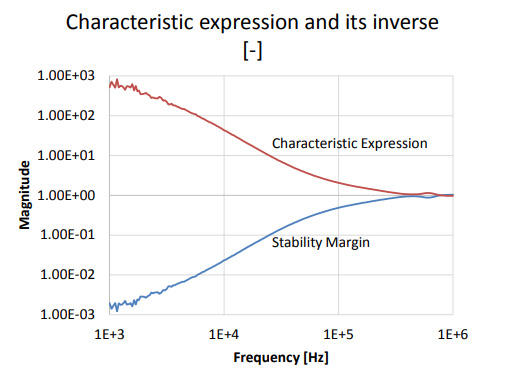Figure 4: Characteristic expression magnitude and its inverse.

For many PDN designs, if we can assume sufficient linearity, the requirement for the output voltage to stay within pre-defined limits gets translated to an impedance target.  Through a few specific examples, this paper explores the possible inter-relation options of loop stability and output impedance.  Though DC-DC converters can be the source of high-frequency noise as well, creating signal integrity and EMC issues , those considerations are outside the scope of this study.  Also not covered in this paper is the considerable percentage of high-current DC-DC converters today that employ nonlinear features in their feedback loop, offering tighter noise control.  In that case impedance-based PDN design is not valid any more, and the same is true for the stability metrics: gain-phase curves and all of their derivatives are not valid for those cases.

### Gain-phase plots

As it was shown in Figure 2, the magnitude and phase of the complex loop gain, as described in Equation (1), can be plotted against a horizontal logarithmic frequency scale and customarily it is called the Bode plot.  The data can be collected by measurement on a live DUT or it can be simulated if sufficiently accurate simulation tools and models are available.

In measurements or simulations, when there is an accessible feedback path monitoring the output voltage, a convenient location to inject the test signal is where the upper leg of the output voltage divider connects to the output.  The simplified schematic in Figure 5 shows such a case; the injection circuit is connected between R1 and Vout.  As long as the injection source impedance is much lower than the R1 impedance loading it, the approximation works well.  The complex loop gain is the Va/Vb ratio of the voltages at the two terminals of the injection circuit.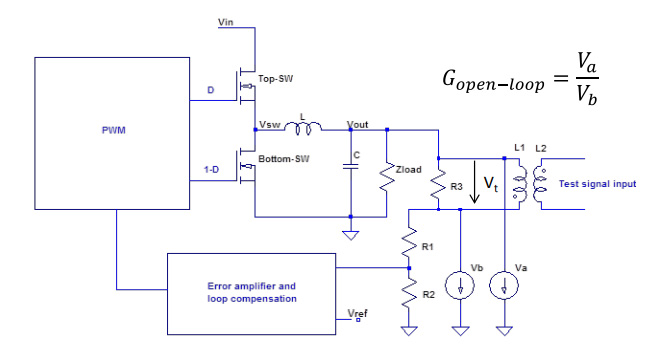Figure 5: Typical connection scheme showing the measurement or simulation setup for gain-phase measurements.

The measurement or simulation done based on the definition shown in Figure 5 is quite robust.  The voltage regulator may be located further away from the load, requiring a longer connection from R1 to Vout.  In such cases the injection circuit around R3 can be anywhere along the connection, we just have to make sure that the Va and Vb voltage measurements (or simulations) reference the same return point, but its exact location does not matter.  As it is shown later, there are still a few things that can go wrong with this setup.  In measurements, selecting the proper injected test signal level is important: the usual loop gain curve may change its magnitude value several orders as we sweep the frequency.  This increases the possibility that the loop amplifier may saturate and clip the signal for too large injection levels or may become too noisy for low injection levels.  Monitoring the measured Va and Vb voltage levels and adjusting the injected signal level helps to avoid this problem.  In simulations, if we use linearized averaged models, saturation is not an issue.  The main inconveniences of this setup are that a) it needs three cables or probes to attach to the DUT, b) we need an isolation transformer to allow us to connect the injected signal to the output voltage DC potential, and most importantly, c) the measurement is invasive: we need to have access to the feedback loop and we have to modify it to inject our test signal.  These inconveniences led to various approximations, described in the next section.

#### Gain-phase plot approximations and non-invasive measurements

There are different ways of measuring the loop gain in approximate, non-invasive ways.  For instance, we can use Equation (2) and calculate the loop gain from the open-loop and closed-loop output impedances.  Output impedance measurements use instruments connected in parallel to the converter output and therefore it is considered to be non-invasive.  Measuring the closed-loop output impedance is often a validation task anyway; we just need to obtain also the open-loop output impedance.

Strictly speaking, obtaining the open-loop output impedance would also require modification to the loop, but sufficient approximations will help.  First, we don’t want to put the voltage regulator completely into open-loop mode, because the output voltage may drift too much due to potentially high DC loop gains.  Second, to assess the stability of the control loop, we don’t need the loop gain (and therefore the open-loop output impedance) in frequency ranges where the loop gain magnitude is either >>1 (typically happens at very low frequencies) or <<1 (typically happens way above the crossover frequency).  In the frequency range where the loop gain matters for stability, the open-loop output impedance can often be approximated with the un-powered output impedance.  These measurements are simple and non-invasive, but we need to ensure that the approximating un-powered output impedance is measured with a DC bias matching the DC output voltage of the powered output impedance measurement.  This is important when high-density ceramic capacitors are used on the output, which can exhibit significant DC (and AC) bias sensitivity. Figure 6 shows an illustration of un-powered output impedance measured with and without the proper DC bias applied. The circuit had a combination of polymer tantalum bulk capacitors and ceramic capacitors.  Note that the impedance magnitude and phase change substantially around 100 kHz due to the DC bias effect of ceramic capacitors.  Further considerations for this approach are shown in Section VI.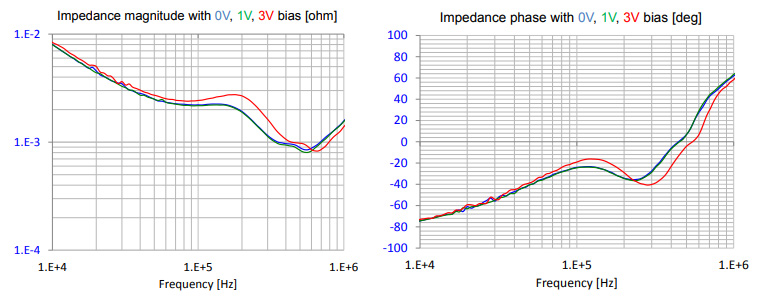Figure 6: Illustration of measuring Gain-phase curves based on open-loop and closed-loop output impedances.

Another possibility is to look at the closed-loop output impedance only.  The output impedance is formed by the output LC filter, the user-defined complex load and the frequency dependent loop gain.  A simplified approximation of the output impedance near the crossover frequency is a second-order LC filter, where the Q (peaking) of the impedance curve is linked to the phase margin.  The non-invasive stability measurement (NISM) is a phase-margin measurement method based on the complex impedance and group delay of closed-loop DUT output impedance .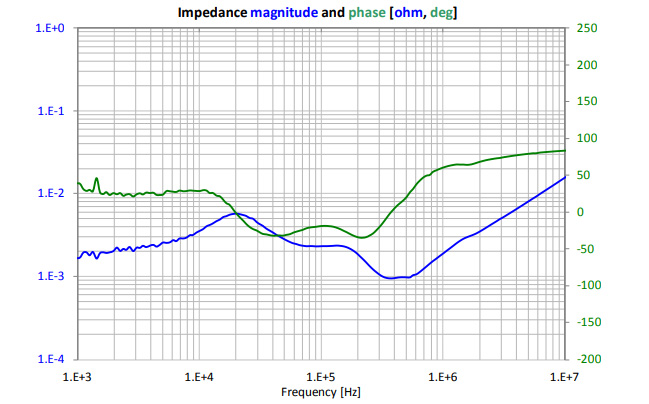Figure 7.a: Illustration of measured impedance magnitude and phase on low-noise DUT.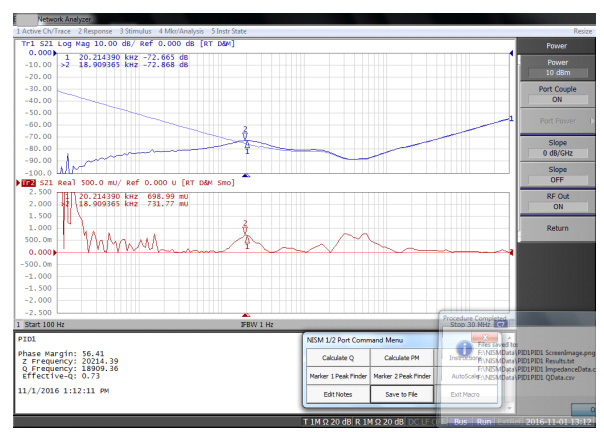Figure 7.b: Illustration of measuring phase margin on low-noise data based on NISM.

The measurement is simple and it uses the output impedance data that the user may also want to collect. The process is limited to cases when there is a measurable peaking in the output impedance (typically phase margins of 70 degrees or lower) and it provides only the phase margin, one data point on the Gain-phase curves.  Also because the process uses the group delay of the measured impedance, which is the derivative of phase, inherently noisy voltage regulators may not be a good candidate for this approach.  Figure 7 shows measured data points on a non-isolated buck regulator with digital PWM controller with different approaches, including NISM.  Note that due to jitter on the main switching edges, though the impedance magnitude (top window, blue traces) looks smooth and clean, the associated group delay curve (lower window, red curve) is noisy.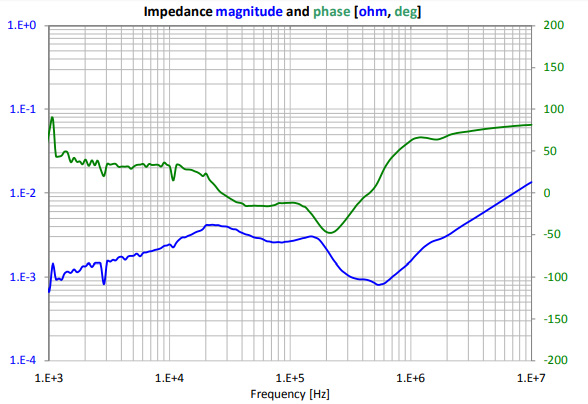Figure 7.c: Illustration of measured impedance magnitude and phase on a noisy DUT.

The impedance magnitude and phase data on the low-noise DUT look clean and smooth and correspondingly the red group-delay curve in the lower window on the NISM data has a smooth and clear peak.  In Figure 7.c, the phase trace of the measured output impedance of the noisy DUT already shows small sharp dips and spikes, which eventually translates to a much noisier group-delay curve in the NISM data in Figure 7.d.

Finally we have to note that any stability measurement based on output impedance data will be sensitive to the location of the measurement (see Section V.4).  This is in contrast to the direct gain-phase measurements, where the location of test points matters very little.Figure 7.d: Illustration of measuring phase margin on noisy data based on NISM.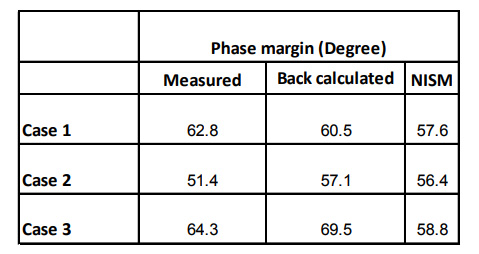Table 1: Comparing phase margin values obtained by direct measurement, back calculated from ON and OFF impedances and measured with NISM.

Table 1 gives a simple comparison overview of phase-margin numbers obtained on the same DUT with three different compensation settings.  The data was obtained by three different approaches.  Note that all three approaches yielded slightly different numbers.  The most robust and most repeatable was the direct measurement, but it requires the most preparation.  The NISM is the simplest to perform, but out of the three approaches shown here, it was the most noise sensitive.

### Nyquist plots and Stability Margin

Nyquist plots provide an additional method to review and asses the stability of a system based on the same open-loop gain data that is used for the gain-phase Bode plots, except it is now plotted on a rectangular real-imaginary pair of axes. Visually, one can approximately see the gain and phase margin, but more importantly, can also see how far from the -1,0 critical point the system is and not just at a gain of 0 dB or at a phase of 0 degree.  The main drawback to the Nyquist plot is that the frequency is not part of the axis but instead is embedded in the data line.  Without additional markers, one cannot tell the frequency associated with specific features on the plot.

Figure 8 shows a combination of data on the same DC-DC converter that was used for Figure 7, intentionally compensated to an operating point with relatively poor stability.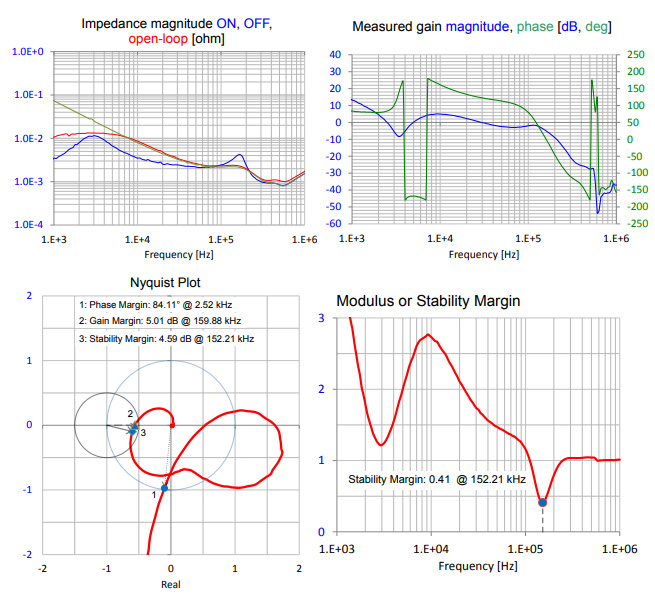Figure 8: Illustration of impedance, gain-phase, Nyquist and Modulus margin plots on a relatively unstable regulator.

The upper left plot shows the impedance magnitude with the converter ON, OFF (with proper DC bias) and overcompensated, to approximate the open-loop impedance at medium and higher frequencies.  The upper right plot shows the Gain-phase Bode plot measured according to Figure 5.  The first zero crossing of the gain magnitude curve occurs at very low frequencies and it comes with an excellent phase margin.  However, the gain magnitude rebounds and crosses zero two more times at higher frequencies. Moreover, after the last zero crossing, the gain remains just barely below zero dB.  All these potential problems are well visible on the Nyquist and Modulus margin plots.  The phase margin (point 1 on the plot) can be found where the red trace crosses the unity circle (corresponding to 0 dB on the Bode plot).  As frequency increases, the trace makes a loop and crosses the unity circle two more times.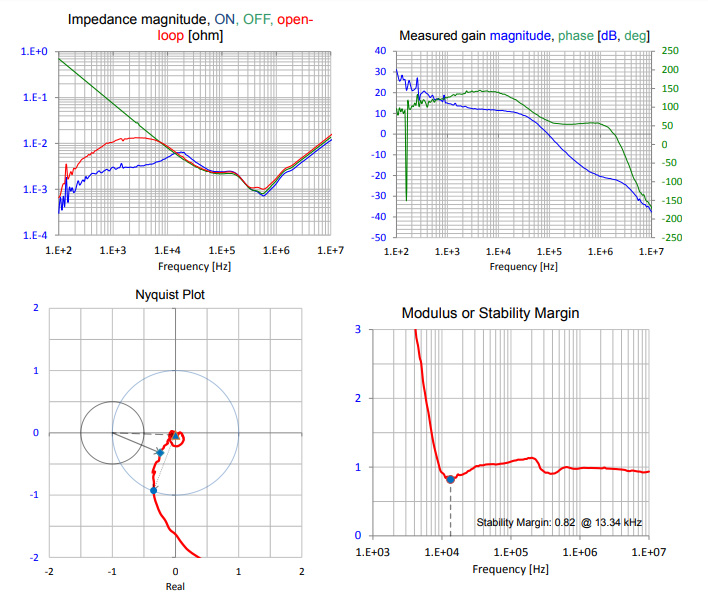Figure 9: Illustration of impedance, gain-phase, Nyquist and Modulus margin plots on a relatively stable regulator.

Going to higher frequencies, we first reach the modulus margin point (point 2), where the distance to the critical point is the shortest.  At somewhat higher frequencies, we reach the gain-margin value (point 3), where the imaginary part is zero (phase equals zero).  The modulus margin is defined as the characteristic expression and its inverse is the sensitivity function .

The measured output impedance, gain-phase Bode plot, Nyquist diagram and modulus margin plots for the same regulator with a better compensation setting are shown in Figure 9.

Note that in both cases, in Figure 8 and Figure 9, the unpowered but properly biased OFF impedance and the open-loop output impedance, approximated by the closed-loop output impedance with a low-frequency dominant pole added, line up very well in the entire frequency range where we can expect the crossover frequency.  The approximate open-loop impedance deviates from its expected value only at lower frequencies, where it gradually approaches the ON impedance.

### Other Metrics

Beyond the absolute minimum requirement of control-loop stability, target-impedance based PDN designs may prefer flattening out the impedance profile, since this minimizes the worst-case transient noise for linear and time-invariant systems.   and its references explain and illustrate the process.

It is also well documented that impedance peaking in the frequency domain can be used to predict non-monotonic transient response.  When we design a PDN and keep the impedance profile below a target value, in the time domain the maximum noise or transient response can be estimated by ΔV=ΔI*Z(f) if and only if the impedance profile is flat enough.  Any non-flatness, even if it deviates from the target value downwards, will increase the worst-case transient noise.  Based on this realization, one simplistic quality metric, directly related also to stability, is to compare the ON and OFF impedances of an active voltage regulator.  Our goal is to make the OFF impedance profile relatively smooth and flat beyond the expected crossover frequency and when we turn on the voltage regulator, we monitor the change in the impedance profile: we consider a regulator loop good if the active loop does not push the impedance above the OFF impedance (or not by much).  We can realize that this is equivalent to indirectly observing the inverse of characteristic expression, also called the sensitivity margin.

An illustration of this is shown in Figure 10, where we show the impedance profile and characteristic expression for the system for which data was shown in Figure 8.  The peaks in the ON impedance profile correlate to the minima in the modulus margin shown in the characteristic expression.  Note also that the visual comparison between the ON and OFF impedances is a very good indicator of the stability margin: when peaks of the ON impedance come close to the OFF impedance, the stability margin trace dips and when the ON impedance exceeds the OFF impedance, the stability margin function drops below one.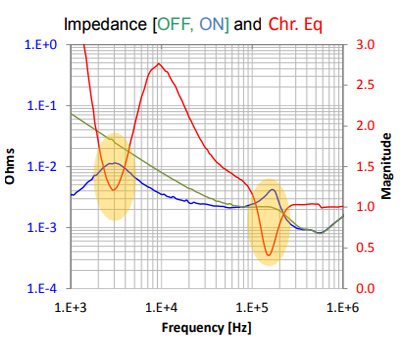Figure 10: Illustration of impedance profile and Characteristic expression

### What Can Go Wrong?

As with any measurement, there are several possible ways how accidentally we can collect wrong or corrupted data.  In this case let us assume that the instrument itself is in good shape, it is properly calibrated and the cables and probes are up to their task.  There are still plenty of opportunities to accidentally get bad data, mostly due to various ways how we make the connections and how we arrange instrument setups.

#### Wrong test signal level

The gain-phase and impedance measurements are done in the frequency domain, though assuming good linearity, they can be derived from time-domain data as well.  In either case, a calibrated sinusoidal signal is injected into the system (a small series voltage when we measure the loop gain, or a current across the converter output when we measure impedance).  The injection level matters more for the loop gain measurements, because the typical converter gain magnitude can vary several orders of magnitudes: too low injection level will bury parts of the response in noise, too high levels may cause saturation somewhere along the loop.  Figure 11 reproduces Figure 42 from .  The top plots are the ratios of the two measured voltages, the bottom plots are the actual readout voltages at the two probe points.  If there is no noise, nonlinearity or saturation, all curves in the top plots should be running perfectly on top of each other.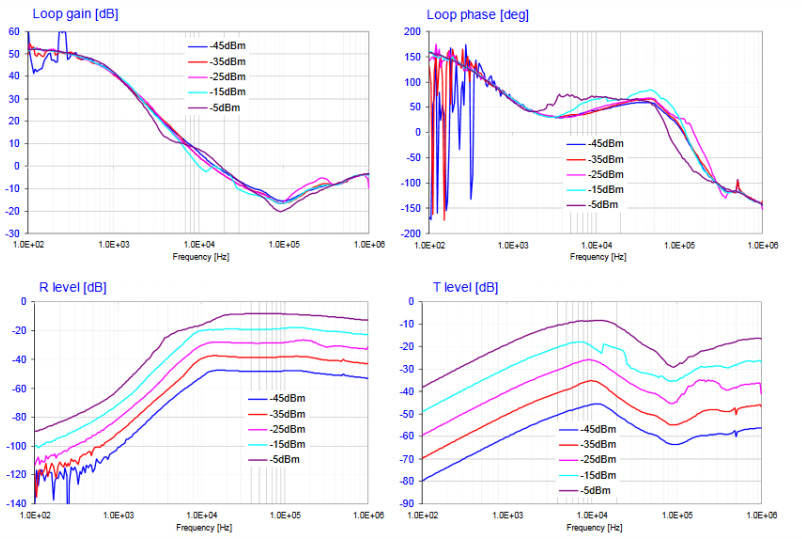Figure 11: Illustration of measured gain-phase curves as a function of injection levels.

The reference channel reading (R) clearly shows signs of noise at low frequencies for injection levels below -30 dBm, and at -15 dBm and above the curves are separated in the 2 – 20 kHz frequency range, which is a clear sign of nonlinearity or saturation.

#### Spurious signals

Gain-phase, impedance, and transfer-function measurements are done in the frequency domain.  A swept-frequency sinewave generator injects a test signal and one or more highly selective tracking receivers process the response(s).  The effective bandwidth can be narrowed down to a few hertz or less, which will reject most of the random noise components. Periodic components, however, which accidentally may coincide with a measurement frequency point, will alter the measurement result.  In switching regulators, the main switching frequency and its harmonics are the most likely sources of such spurious signals.  Since we should know the main switching frequency, or if we don’t, it is easy to measure, it makes it easy to handle such cases. If an outlier data point shows up in the frequency domain data, we can either ignore that data point, or we change the frequency sweep parameters such that no measurement point will fall on the switching frequency.  Sometimes, on the other hand, spurious signals may also show up at unpredictable frequencies, not related to the main switching frequency.  The following figures illustrate such cases.  Figure 12 shows the measured output impedance of a small switching regulator at two operating points.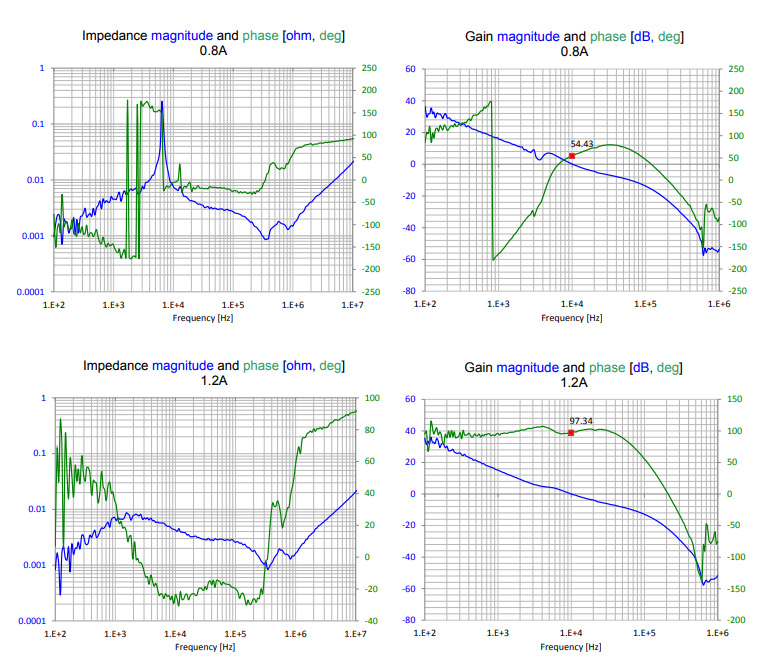Figure 12: Measured output impedance and gain-phase curves of a small buck converter with 0.8A and 1.2A DC load current.

The DC load current is 0.8A and 1.2A on the top and bottom plots, respectively.  Plots on the left show impedance, on the right the gain-phase plots.  The red dot and the numeric marker on the gain-phase plots identify the phase margin.  The input voltage was 13.5V for all cases, the output voltage was fixed at 1.05V.  The converter was measured at a large number of operating-point permutations by stepping the input voltage and DC load current in small increments over their full allowed ranges.

At many operating points, the response was very similar to what we see here with the 1.2A DC load and only a small number of operating points, all of them clustered at the high input voltage and around the same current value.  The bottom impedance and gain-phase curves with 1.2A load look normal, but at 0.8A load there is a large peak in the impedance profile and a much distorted phase curve and lower phase margin in the gain-phase plot.

The sharp peak in the impedance magnitude does not appear to come from the converter’s main switching signal for two reasons. First, its frequency is 6.2 kHz, while the switching frequency was 600 kHz and changing the switching frequency had little effect on the peak frequency.  (Not documented here, but it was also noticed that the frequency of the peak did change if a test signal attempted to excite it.)  Second, the peak has a widened base with an overall shape that is more characteristic to circuit resonances.  We can also notice that at 0.8A load current, three out of the four plotted parameters look unrealistic: the impedance magnitude and phase and the phase of the loop gain.  The gain magnitude curve shows very little disturbance.  Further analysis was necessary to reveal the reason.  First it was confirmed that the large impedance peak means that the time-domain response signal gets big.  Figure 13 shows the waveform at the output of the converter.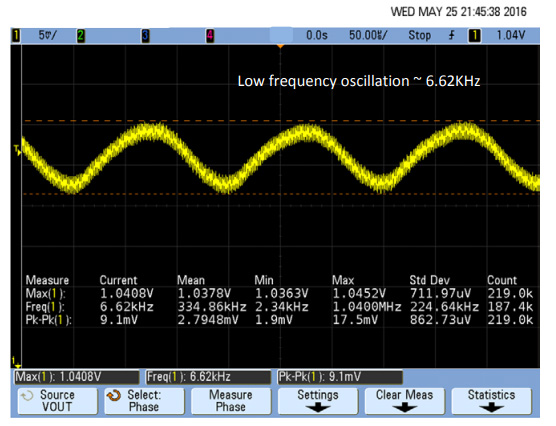Figure 13: Low-frequency periodic disturbance on the converter output.

With 0.8A DC load, there is a 9 mVpp periodic fluctuation with the same frequency where the impedance peak occurs. When the oscilloscope and the network analyzer measuring the impedance were connected to the converter output simultaneously, the oscillation frequency on the oscilloscope followed the network analyzer sweep over a small frequency range.  This frequency range seems to be the same as the widened base of the impedance peak. To correlate the measured impedance to the transient noise magnitude, impedance measurements were done with different test-current levels.  A transient tester circuit was pre-biased to Class-A operating point, and the swept sinewave output of the network analyzer modulated the DC current.  The source power of the network analyzer was stepped through a large range so that the injected test current level varied from milliamperes to amperes.  The 3D surface of Figure 14 shows the result.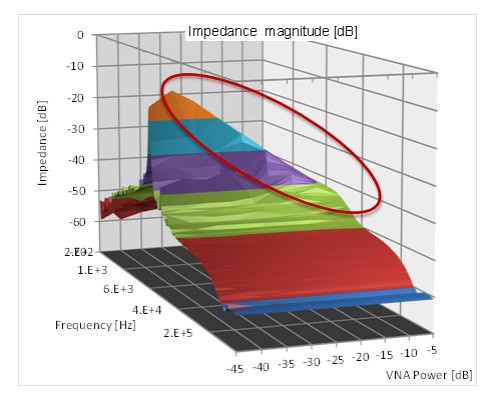Figure 14: Measured output impedance with different levels of test signals.

The plot is truncated to show only the important portion of the frequency range where the peak occurs.  Note that almost in the full range of input levels the measured peak impedance follows a proportional straight ridge line: higher injection level means lower impedance peak.  As a result, the calculated noise, which is impedance multiplied by current, stays the same.  It turns out that this value is approximately the level of the spurious signal, which means that the network analyzer measured the spurious signal instead of the response to its own excitation.  The narrow bandwidth of the network analyzer did not help, because over multiple test-frequency points, the spurious signal locks to and tracks the network analyzer’s test signal.

In general, with spurious periodic signals present in the DUT, the frequency domain measurements may become meaningless, or at least the proper interpretation of the result becomes more difficult.

#### Impact of test equipment and load circuit

The simple equivalent circuit of Figure 1 may be modified in practical circuits by several factors.  On a fully populated system board without proper initialization, the load device’s impedance may be unknown.  This potential problem can be eliminated if we depopulate the current-consuming loads and replace them with electronic loads that we control.  Modern electronic loads usually offer a choice for the impedance they present: constant-current mode, constant-resistance mode, or constant-power mode.  In constant-current mode we assume that the impedance of the electronic load is close to infinite.  This may be true at DC, but not at higher frequencies.  The output of high-current electronic loads have some capacitance added intentionally across the output terminals.  Together with the potentially long cables we have to use to connect to our DUT, we can get a strongly frequency-dependent impedance.  Figure 15 shows the measured impedance of an electronic load after 60” long connecting cables.  The instrument was unpowered, so the impedance reflects the passive components across the output.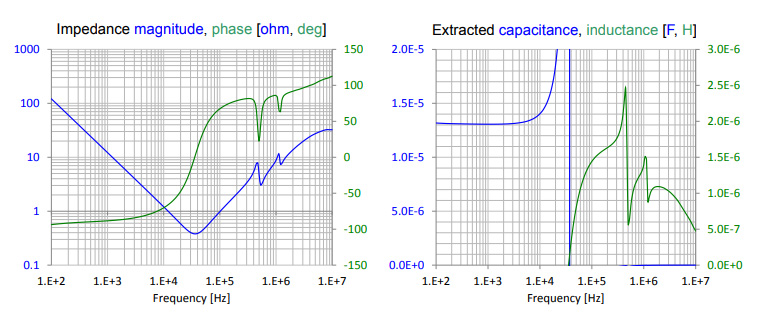Figure 15: Measured impedance of a 300A electronic load in OFF state: impedance magnitude (blue) and phase (green) on the left and extracted capacitance (blue) and inductance (green) on the right.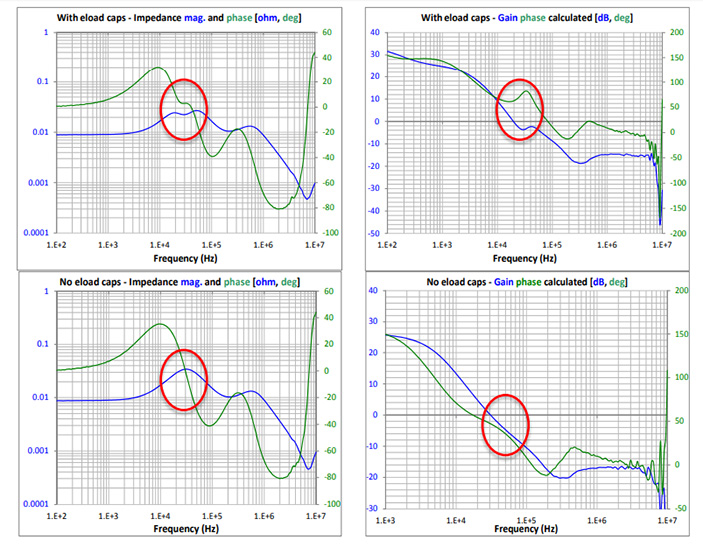Figure 16: Impact of the electronic load on the converter measurement.

This can easily happen in situations when secondary filters are used to further lower the switching ripple of the converter output.  If the filter cutoff frequency is conveniently set below the switching frequency, it may come close or could coincide with the crossover frequency.

As a general rule, we may aim to separate these natural frequencies, but lowering the secondary filter’s cutoff frequency further will require a bigger inductor or capacitor, or both, so eventually it may become a size and/or cost problem.  Figure 17 compares the ON and OFF impedances of a POL converter with and without the secondary filters connected to the output.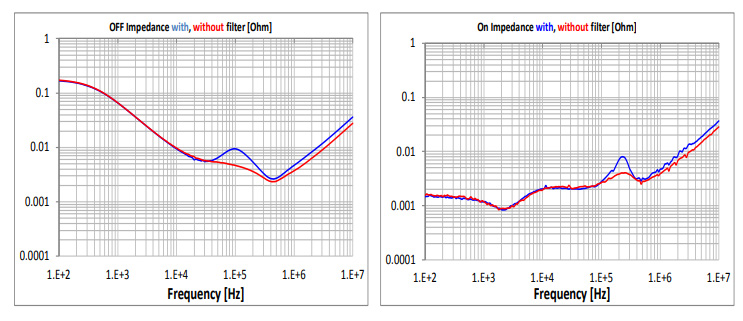Figure 17: Buck converter impedance with and without secondary filters.

A similar effect can occur when the secondary filtering happens by accident, not by intention.  When the series plane resistance interacts with thousands of microfarads of bulk capacitance, a low-pass filter is created.  For instance, one milliohm of plane resistance and 10,000 uF bulk capacitance produces a 16 kHz cutoff frequency, which creates substantial phase shifts in the typical crossover frequency range of DC-DC converters.  Figure 18 is data reproduced from , which illustrates such a scenario.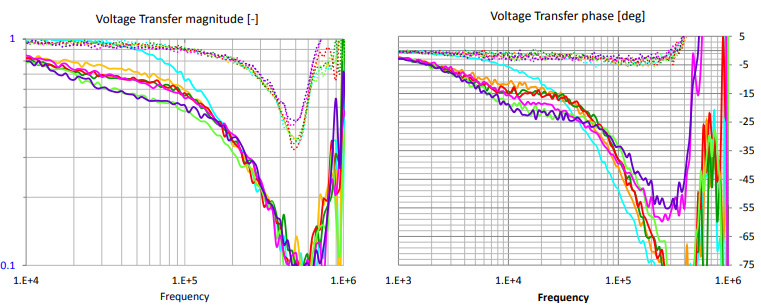Figure 18: Impact of the cutoff frequency created by the lane resistance and bulk capacitance: magnitude of voltage transfer on the left and phase of the voltage transfer on the right.

The sketch of the geometry is reproduced in Figure 19.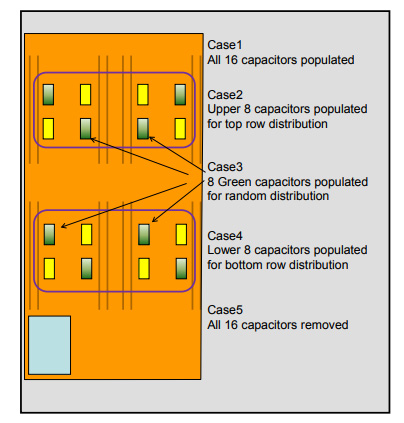Figure 19: Sketch explaining the various configurations.

#### Measurement Location

To measure the open-loop gain directly, we can insert a test signal somewhere along the loop where a low-impedance point drives a high-impedance circuit.  Such a location is, for instance, where the voltage feedback loop senses the output voltage.  The output is typically a low-impedance point and the sense circuit has much higher impedance.  The sense circuit can be a high-impedance voltage-monitor input without a voltage divider, or a voltage divider stepping the output voltage down to the level of the internal voltage reference, as shown in Figure 20.  If this point is accessible, we can insert a test signal through an isolation transformer.  Note that to maintain the DC operating point, we do not want to physically cut the loop open, rather we measure the ratio of the complex voltages on the two sides of the injected test signal. A similar connection can be applied to any power converter, including analog voltage regulators as well.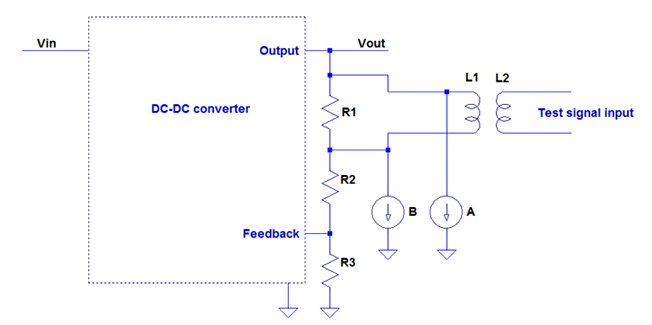Figure 20: Block schematics of test signal injection.

Some integrated converters have the voltage-sense feedback or the upper leg of the voltage divider inside the module, or the accessible feedback path has only high-impedance points.  In such cases. different connection methods have to be used, see for instance .  Nevertheless the basic concept remains the same: we inject a small test voltage into the control loop and measure the ratio of two response voltages.

When the scheme in Figure 20 is used, we have to connect three cables to the DUT.  The signal injection cable is floating and the other two cables reference the DUT return.  If the voltage sense is further away from the controller IC, we can inject the test signal anywhere along the trace leading to the point where it connects to the output rail and since the open loop gain is measured as the ratio of the voltages at the two connection points of the test-signal injection, the location of cable returns is usually not critical.  However, we need to make sure that the two cable returns are connected to the DUT main return at about the same location.  When we use output-impedance information to calculate phase margin or calculate the full gain-phase plot, we have to make sure that the measurement location is where the converter’s sense point connects to the output.  Figure 21 illustrates what happens if the connections are at different points.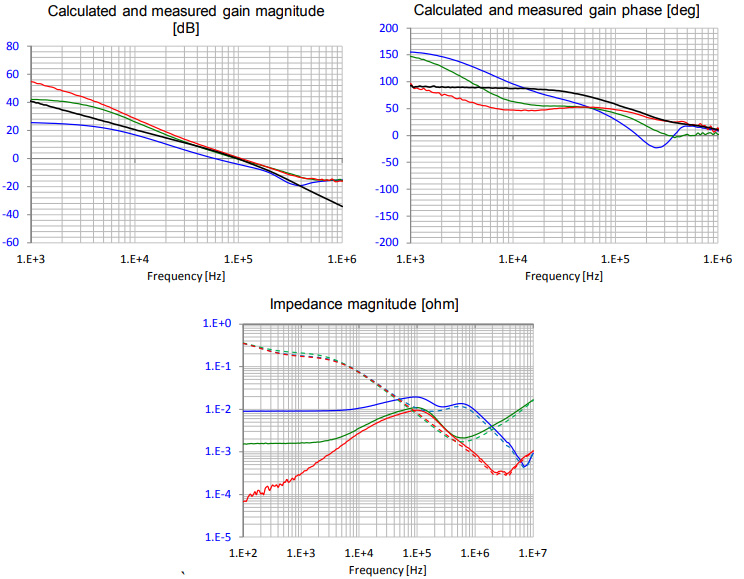Figure 21: Impact of connection location on the gain-phase magnitude (top left) and back-calculated gain-phase phase curves (top right) and measured impedance (bottom).

The DUT was an LDO, with a maximum rated current of 1.5A.  The input and output voltages were 5V and 1.89V, respectively, the data is shown at 0.5A DC load current. The sketch of the circuit layout and the measurement points are identified in Figure 22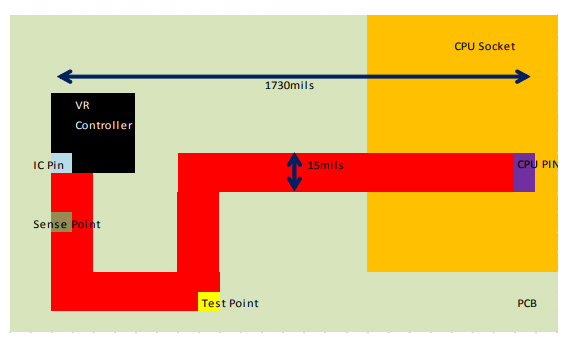Figure 22: Sketch of the DUT layout with measurement points identified for Figure 21.

Figure 23.a shows the output impedance curves measured in the same operating point at three different locations.  The schematic details showing the measurement and feedback-point locations for the three cases are shown in Figure 23.b.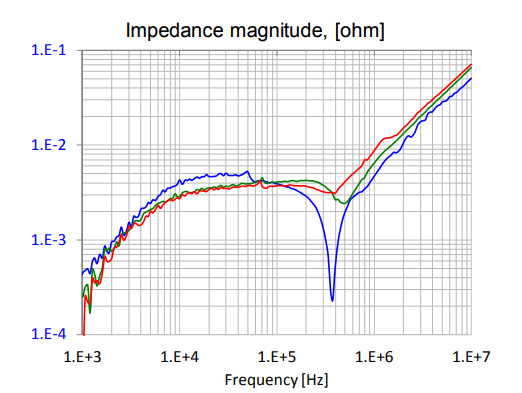Figure 23.a: Measured gain-phase curve at three different locations.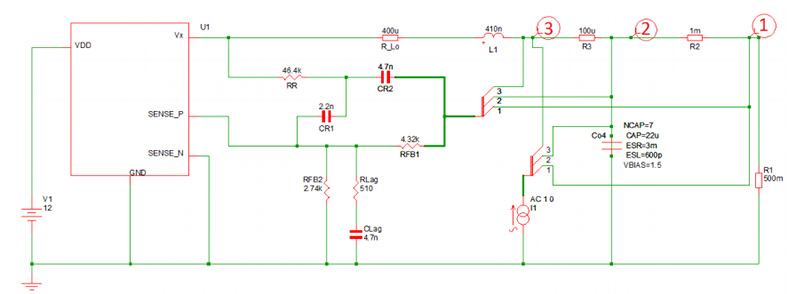Figure 23.b: Approximate schematics indicating the three locations for measurements and feedback-point connection.

Note the difference around the series resonance frequency of the output ceramic capacitors.  The middle curve (green trace) corresponds to the dip measured without power applied.  With the active control loop, the dip minimum impedance either goes up or down, dependent on where the connections is made.

### Conclusions

Various metrics and test methodologies have been looked at and compared for measuring the stability of voltage regulator feedback loops.  It was found that the measurement technique based on the original three-cable gain-phase setup was the most intrusive, but least sensitive to noise and measurement locations.  Approximation of the phase margin and gain-phase curve from unpowered and powered output impedances offers simpler measurements at a price of higher sensitivity to noise and location of measurement connections.

It was also shown that frequency-domain stability and impedance measurements may become invalid or hard to implement when spurious periodic signals in the frequency range of measurement are generated by the DUT.

An earlier version of this paper was a DesignCon 2017 Best Paper Award Winner.

### Acknowledgement

The authors wish to thank Gustavo Blando for his encouragement and suggestions and Steve Sandler, Picotest, for his valuable comments and feedback.

### References

        Christophe P. Basso: Switch-Mode Power Supplies – SPICE Simulations and Practical Designs.  McGraw-Hill, 2008.

        “Dynamic Characterization of DC-DC Converters,” TecForum, Proceedings of DesignCon 2012, January 30 – February 2, 2012, Santa Clara, CA.

        Steve Sandler, "Killing the Bode Plot," DesignCon 2016, January 19-21, 2016, Santa Clara, CA.

        Larry D. Smith, Raymond E. Anderson, Douglas W. Forehand, Thomas J. Pelc, and Tanmoy Roy, ‘‘Power distribution system design methodology and capacitor selection for modern CMOS technology’’, IEEE Transactions on Advanced Packaging, vol. 22, no. 3, pp. 284-291, Aug.1999.

        “Mid-Frequency Noise Coupling between DC-DC Converters and High-Speed Signals,” DesignCon 2016, Santa Clara, CA, January 19 - 21, 2016

        “Dynamic Characterization of DC-DC Converters,” DesignCon 2012, Santa Clara, CA, January 30 - February 2, 2012

        “DC and AC Bias Dependence of Capacitors Including Temperature Dependence,” DesignCon East 2011, Boston, MA, September 27, 2011

        Non-invasive Stability Measurement, https://www.picotest.com/non-invasive-stability-measurement.html

        Christophe P. Basso: Designing Control Loops for Linear and Switching Power Supplies.  Artech House, 2012.

      “Systematic Estimation of Worst-Case PDN Noise,” http://www.electrical-integrity.com/Quietpower_files/QuietPower-34.pdf

      “How to Measure the Loop Transfer Function of Power Supplies,” Texas Instruments Application Note, AN-1889, SNVA364A, October 2008, Revised April 2013

      “Impact of Regulator Sense-point Location on PDN Response,” DesignCon 2015, Santa Clara, CA, January 27 - 30, 2015

### Author(s) Biography

Joseph ‘Abe’ Hartman is a Principal Hardware Engineer focusing on system signal and power integrity at Oracle. Abe has worked as a signal integrity engineer at Amphenol TCS, Juniper Networks, and Enterasys. Abe also worked at General Motors. Abe holds a MS in Electrical Engineering from the University of Massachusetts-Lowell, a MS in Engineering Science from Rensselaer Polytechnic Institute, a BS in Mechanical Engineering and a BS in Electrical Engineering from Kettering University, Flint, MI.

Alejandro ‘Alex’ Miranda is a Senior Hardware Engineer at Oracle focused in power integrity for SPARC Low/Mid range servers. Prior to joining Oracle, Alex was a Hardware Engineer at Intel working on the design of DC/DC converters and PDN simulation for datacenter products. Alex earned his BS in Electrical Engineering from Instituto Tecnologico de Celaya, Mexico

Kavitha Narayandass is a Hardware Engineer at Oracle. Her work focuses on Power Integrity for SPARC servers. Prior to joining Oracle, she was a Hardware Design Engineer, working on Circuit Design and Signal Integrity simulation at Wipro Technologies, India. She holds a Bachelor's in Electrical and Electronics Engineering from Bharathiar University, India and is pursuing her Master's degree in Electrical and Computer Engineering at Georgia Institute of Technology, GA

Alexander Nosovitski is a Principle Hardware Engineer at Oracle with over 15 years experience in interconnect modeling and characterization, RF design and system simulation. Currently he works on system modeling, simulations and validation on Oracle’s server platforms. He received his B.S. in Electrical and Computer Engineering from Northeastern University and M.S. in Software Engineering from Brandeis University.

Istvan Novak is a Senior Principle Engineer at Oracle and an editioral advisory board member of the Signal Integrity Journal. Besides signal integrity design of high-speed serial and parallel buses, he has been engaged in the design and characterization of power-distribution networks and packages for mid-range servers. He creates simulation models, and develops measurement techniques for power distribution. Istvan has twenty plus years of experience with high-speed digital, RF, and analog circuit and system design. He is a Fellow of IEEE for his contributions to signal-integrity and RF measurement and simulation methodologies.